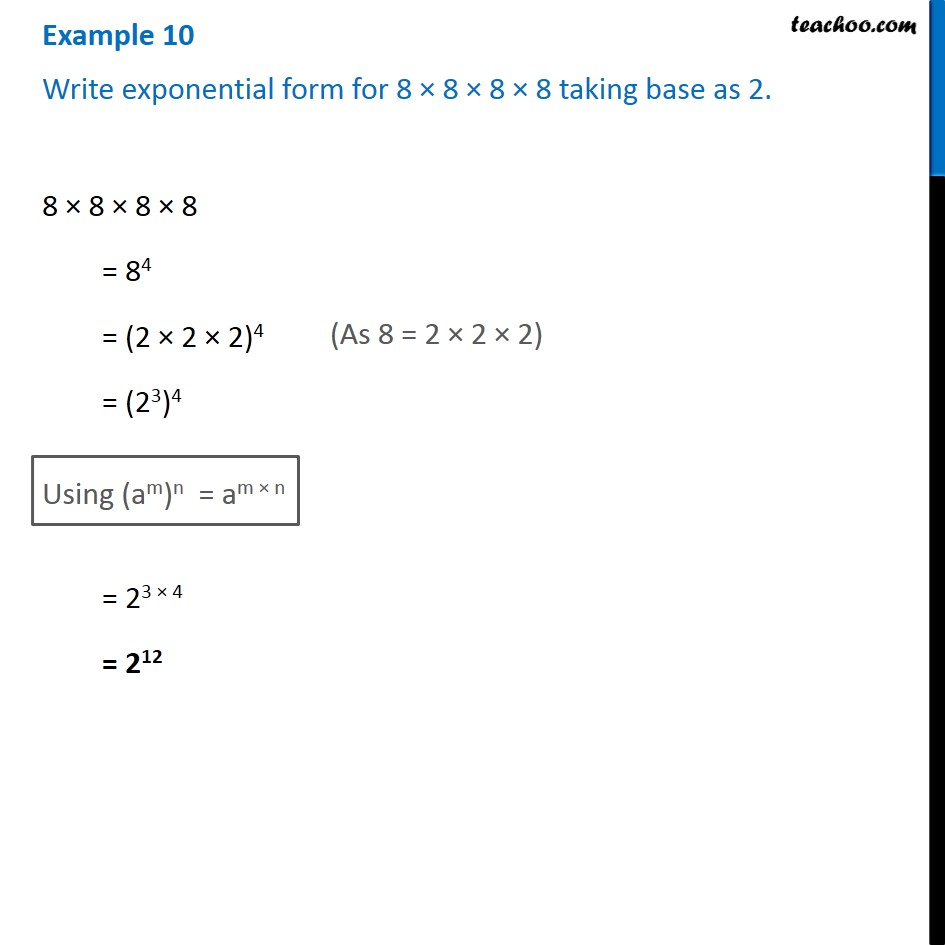1. Chapter 13 Class 7 Exponents and Powers
2. Concept wise
3. Law of exponents

Transcript

Example 10 Write exponential form for 8 × 8 × 8 × 8 taking base as 2. 8 × 8 × 8 × 8 = 84 = (2 × 2 × 2)4 = (23)4 (As 8 = 2 × 2 × 2) Using (am)n = am × n = 23 × 4 = 212